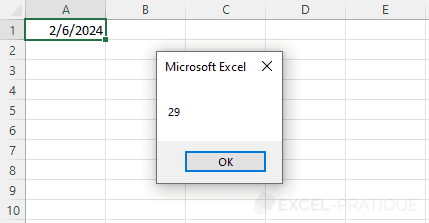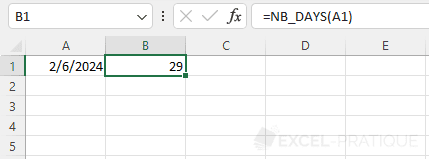# VBA Tip: Number of Days in a Month

You can use the following formula to calculate the number of days in a month or determine the last day in a month:

``````Sub nbDaysMonth()

testDate = CDate("2/6/2024") 'Any date will do for this example

nbDays = Day(DateSerial(Year(testDate), Month(testDate) + 1, 1) - 1)

End Sub``````

Here is a version of the formula broken down into its constituent parts with commentaries that will help you understand how it works:

``````Sub nbDaysMonth()

'Any date will do for this example
testDate = CDate("2/6/2024")

'Month / Year of the date
varMonth = Month(testDate)
varYear = Year(testDate)

'Calculation for the first day of the following month
dateNextMonth = DateSerial(varYear, varMonth + 1, 1)

'Date of the last day
lastDayMonth = dateNextMonth - 1

'Number for the last day of month (= last day)
nbDays = Day(lastDayMonth)

End Sub``````

## Function

You can also use the above code as a function by adding the following code to a module:

``````Function nbDays(testDate As Date)
nbDays = Day(DateSerial(Year(testDate), Month(testDate) + 1, 1) - 1)
End Function``````

Example of how the function can be used in VBA:

``````Sub example()
test = nbDays(Range("A1"))
MsgBox test
End Sub``````Example of how the function can be used on a calculation worksheet: